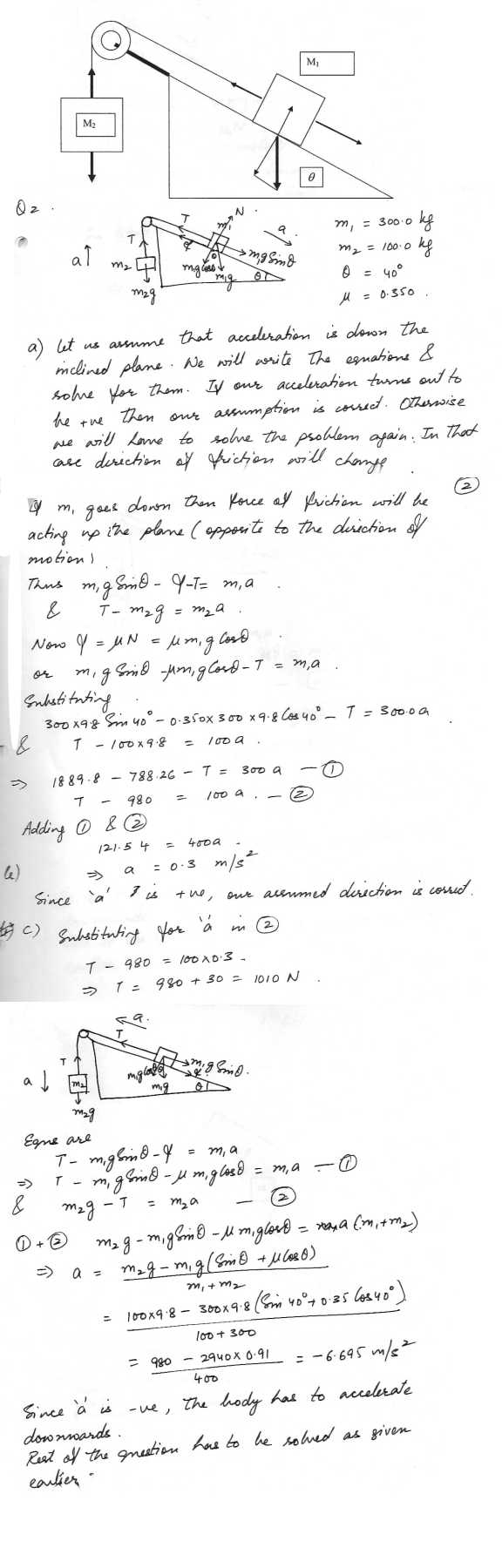58

Physics Force & Laws Of Motion Level: High School

A rocket exhausts fuel with a velocity of 1500 m/s , relative to the rocket. It starts from rest in outer space with fuel comprising 80 % of the total mass. Find its speed when all the fuel has been exhausted.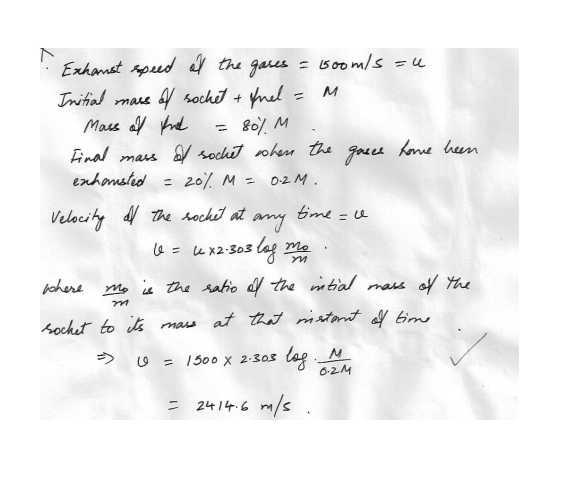57

Physics Force & Laws Of Motion Level: High School

A 500 kg sack of coal is dropped on a 2000 kg flatcar which was initially moving at 3 m/s as shown. Find the speed of the flatcar after the sack rests on it.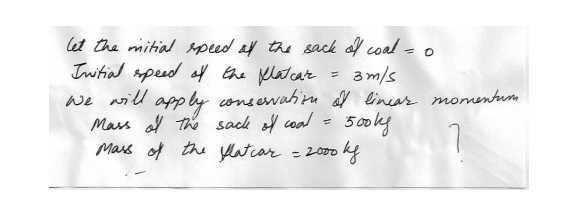56

Physics Force & Laws Of Motion Level: High School

A short 10 gram string is used to pull a 50 gram toy across a frictionless horizontal surface. If a 3.0 x 10 Newton force is applied horizontally to the free end. Find the force of the string in the toy, at the other end.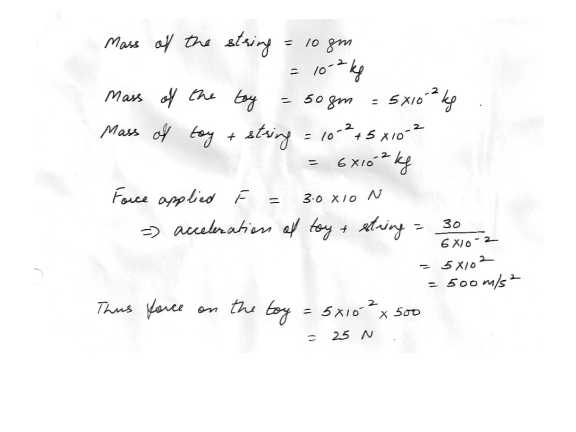55

Physics Force & Laws Of Motion Level: High School

In the figure as shown in "click here", a block slides along a track from one level to a higher level after passing through an intermediate valley. The track is frictionless until the block reaches the higher level. There a frictional force stops the block in a distance 'd'. The blocks initial speed v0 is 7.6 m/s; the height difference 'h' is 1 m; and the coefficient of the kinetic friction 'micro k' is 0.51. Find 'd' .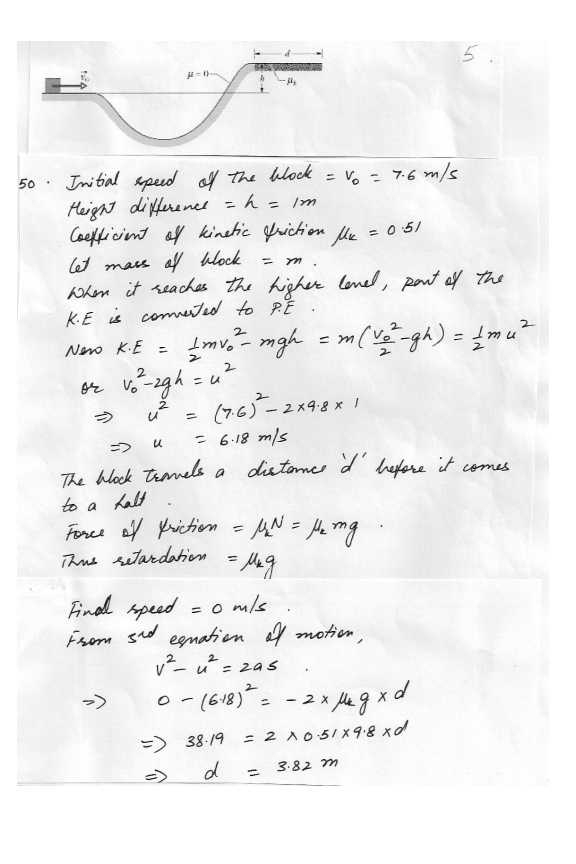54

Physics Force & Laws Of Motion Level: High School

A child whose weight is 287 N slides down a 6.6 m playground slide that makes an angle of 41 degree with the horizontal. The coefficient of the kinetic friction between slide and child is 0.86.

(a) How much energy is transferred to the thermal energy ?

(b) If she start at the top with a speed of 0.568 m/s , what is her speed at the bottom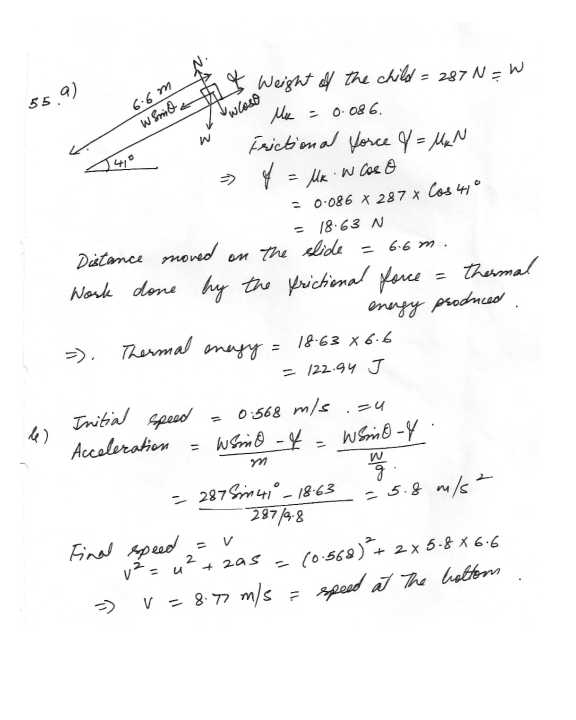53

Physics Force & Laws Of Motion Level: High School

A hockey layer pushes a puck (M= 0.25 kg) initially at rest, with a constant force of 6.0N parallel to the ice. The force acts through a distance of 0.50m. Find the kinetic energy of the puck as it leaves the stick. How much work is required to bring the puck to rest ? what impulse does the hockey stick impart to the puck ?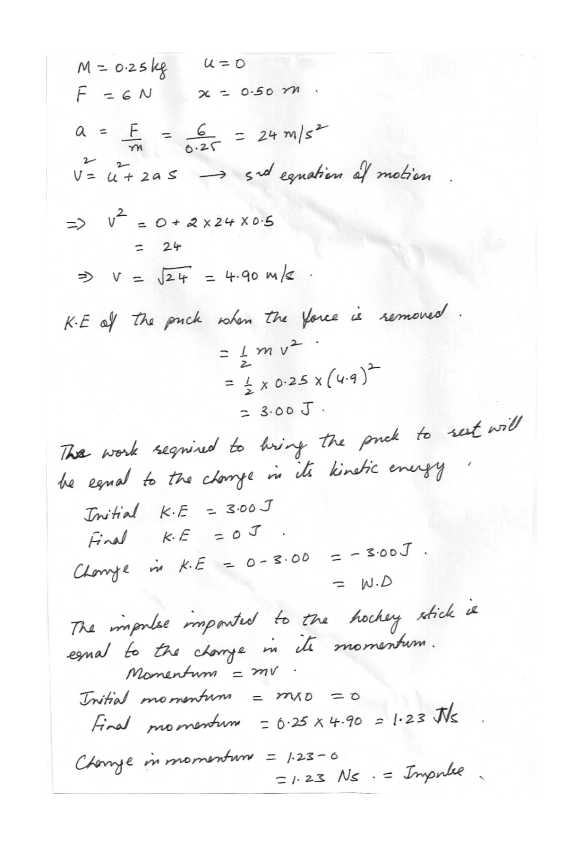52

Physics Force & Laws Of Motion Level: High School

Consider a 22 caliber bullet, a large cruise ship, and an iceberg. Assuming each to move at its normal speed, which has the greatest linear momentum ? Begin by estimating the mass and the speed of each.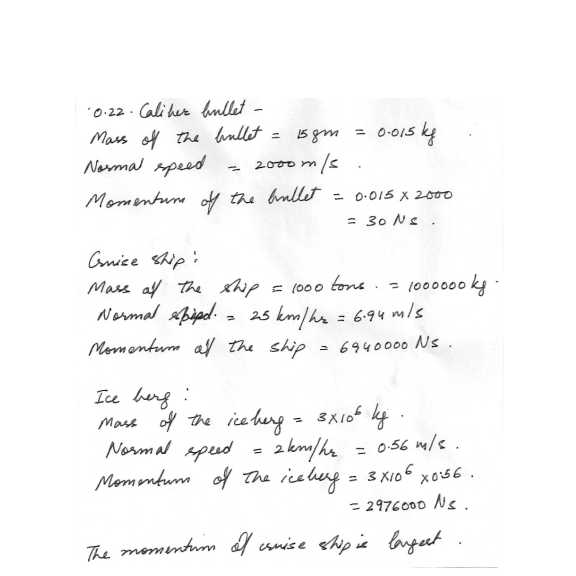51

Physics Force & Laws Of Motion Level: High School

A bullet of mass 100 g is fired horizontally into a 14.9 kg block of wood resting on a horizontal surface, with the bullet becoming embedded in the block. If the muzzle velocity of the bullet is 250 m/s , what is the velocity of the block containing the bullet immediately after the collision ? If the coefficient of the friction between the block and the surface is 0.60. how far will the block slide before coming to rest ?50

Physics Force & Laws Of Motion Level: High School

A gong is suspended by two ropes . One makes an angle of 25 degrees with the vertical and is under tension of 70 N . The other rope makes an angle of 40 degrees with the vertical. Find the mass of the gong and the tension in the second rope ?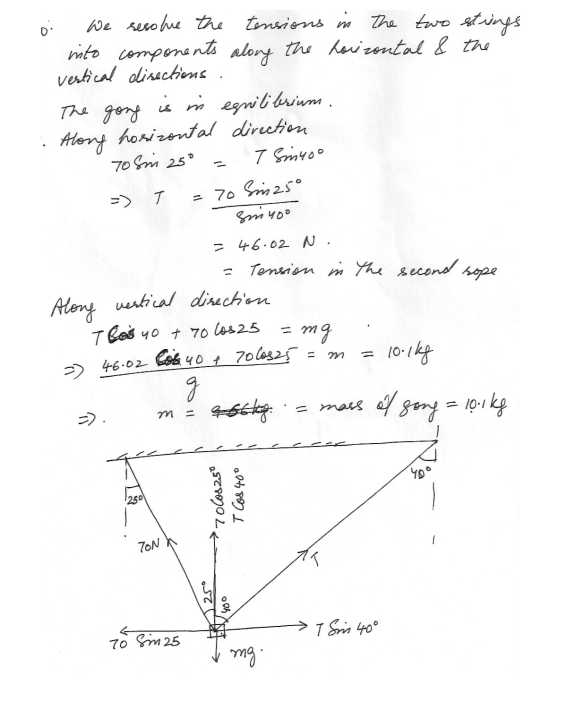49

Physics Force & Laws Of Motion Level: High School

A 15 gram bullet is fired into a 10 kg wounded box which is mounted on small, frictionless wheels. Before the shot, the block is at rest. After the bullet hits the block, the combination of the two masses travels 45 cm in 1 second.

a) Calculate the velocity of the bullet before it hits the block.

b) What impulse is delivered to the block by the bullet ?

c) How much work was done on the block by the frictional forces exerted between the bullet and the block ?

d) How much work was done on the bullet by the frictional forces exerted between the bullet and the block ?

e) Is kinetic energy conserved in this solution ? Give numerical evidence.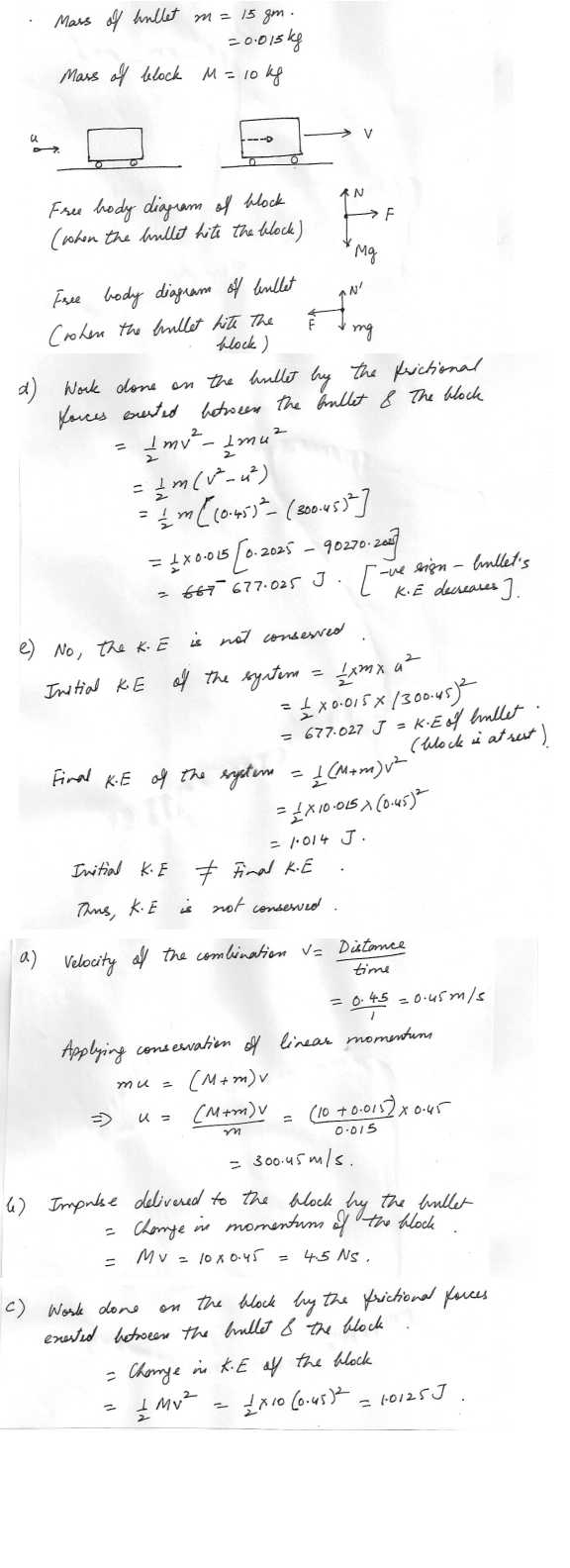48

Physics Force & Laws Of Motion Level: High School

A bicycle and rider together weigh 186 lbs . when moving at 10 mi/hr on a level road, the rider ceases to pedal and observes that she comes to a stop after traveling 200 yds.

a) How much work was done by the frictional force ?

b) What was the average frictional force resisting her motion ?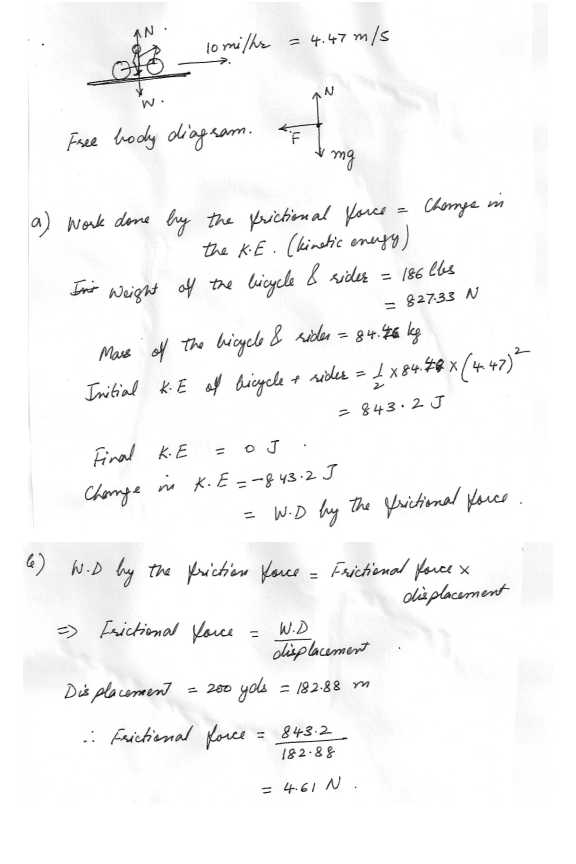47

Physics Force & Laws Of Motion Level: High School

A certain horse says, "There is no use trying to pull that cart. No matter how hard I pull on cart, -it will always pull back on me with exactly the same force. So I can never make it go , " Explain what is wrong with the horse's reasoning. (Free-body diagrams ,showing all the forces involved , will help )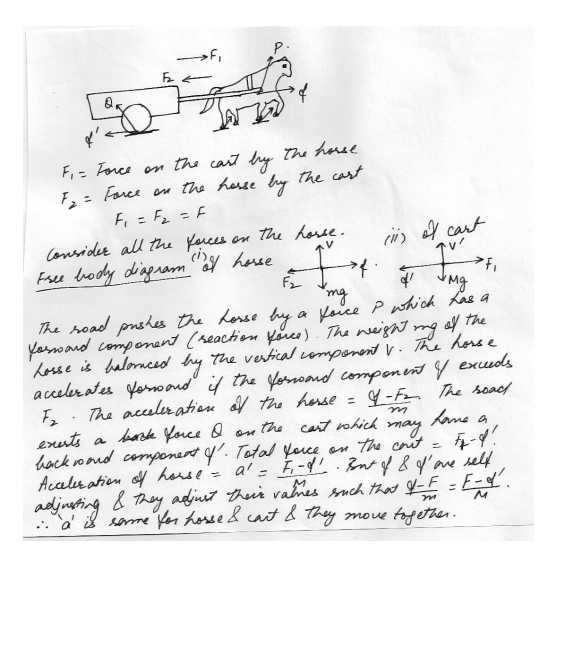42

Physics Force & Laws Of Motion Level: High School

A 15.0 kg uniform board 2.4 m long is pivoted so that it can rotate at a horizontal axis through the end (point A). If a 100-kg mass and is suspended 1.80 m from the pivoted end, what is the tension in the string ?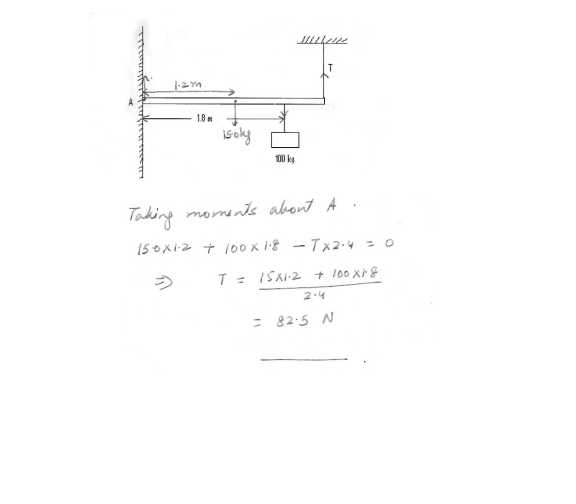41

Physics Force & Laws Of Motion Level: High School

Two masses are suspended from a frictionless pulley as shown in "click here " The pulley itself if has a mass of 10.kg and its radius r = 10 cm.

(a) what is the magnitude of the acceleration of the suspended masses is m1 = 8 kg

(b) what are the tension in the strings? (Neglect the mass of the string.)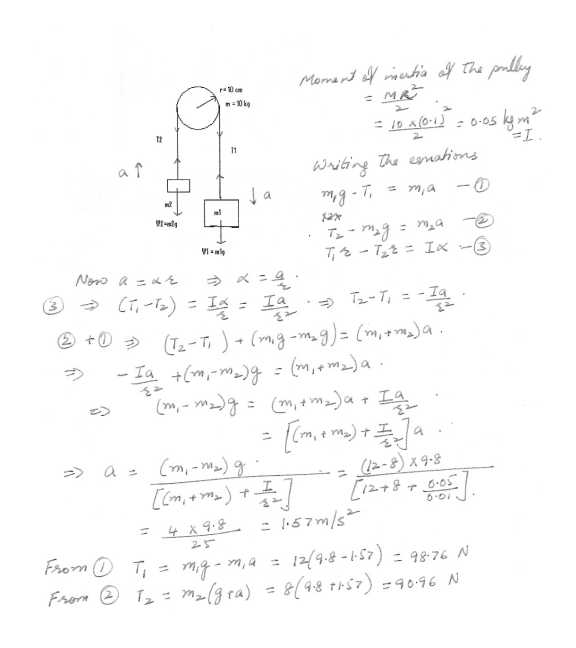40

Physics Force & Laws Of Motion Level: High School

Two blocks are connected by a cable via a pulley which are massless and frictionless. Given the following: m=300.0 kg, m2= 100.0 kg, theta = 40.0 degree, micro = 0.350, determine:

a. The direction of acceleration (mathematically no guesses)

b. The value of acceleration

c. The tension in the cable